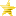Any code for Guard Push? and one more question (Read 175 times)

Started by ziaker, May 01, 2019, 07:02:14 pm

ziakerAny code for Guard Push? and one more question
#1  May 01, 2019, 07:02:14 pm
•••I would like to use code similar to the Guard Push of Skullgirls OR Darkstalkers.

I would also like to know how to make the animation change in my clash hits so when both attacks collide, both of them switch animation as they are pushed by the vel?

Here the CLash HIt code:

Code:
`;Clash[Statedef 12000]Type = SPhysics = N;Anim = 12000;Sprpriority = 0[State 0, Statetypeset]Type = Statetypesettrigger1 = Root, Movetype = IMovetype = IIgnorehitpause = 1[State 0, Statetypeset]Type = Statetypesettrigger1 = Root, Movetype = AMovetype = AIgnorehitpause = 1[State 0, AssertSpecial]type = AssertSpecialtrigger1 = 1flag = invisibleignorehitpause = 1[State 0, ChangeAnim]type = ChangeAnimtrigger1 = 1value = Root, Animelem = Root, Animelemno(0)ignorehitpause = 1[State 0, NotHitBy]type = NotHitBytrigger1 = 1value = SCA,NA,SA,HA,NP,SP,HP,NT,ST,HTtime = 1ignorehitpause = 1[State 0, ReversalDef]type = ReversalDeftrigger1 =  1reversal.attr = SCA,NA,SA,HA,NP,SP,HP,NT,ST,HTsparkno = -1hitsound = S1,0p1stateno = 12001;p2stateno =ignorehitpause = 1[State 0, BindToRoot]type = BindToRoottrigger1 = 1time = 1;facing = 0pos = 0,0ignorehitpause = 1;persistent =[State 0, Turn]type = Turntrigger1 = facing != Root, Facingignorehitpause = 1;persistent =[state 0 destroyself]Type = Destroyselftrigger1 = Root, Stateno != [200,799]ignorehitpause = 1;-------------------------------------------------------------------------------; Clash Helper reversed[Statedef 12001]Type = SPhysics = N;Anim = 12000;Sprpriority = 0[State 0, Statetypeset]Type = Statetypesettrigger1 = 1Movetype = IIgnorehitpause = 1[State 0, Statetypeset]Type = Statetypesettrigger1 = Root, Movetype = AMovetype = AIgnorehitpause = 1[State 0, AssertSpecial]type = AssertSpecialtrigger1 = 1flag = invisibleignorehitpause = 1[State 0, ChangeAnim]type = ChangeAnimtrigger1 = 1value = Root, Animelem = Root, Animelemno(0)ignorehitpause = 1[State 0, NotHitBy]type = NotHitBytrigger1 = 1value = SCA,NA,SA,HA,NP,SP,HP,NT,ST,HTtime = 1ignorehitpause = 1[State 0, Pause]type = Pausetrigger1 = !timetime = 8;movetime = 1;endcmdbuftime = 0;pausebg = 1ignorehitpause = 1;persistent =[State 0, EnvShake]type = EnvShaketrigger1 = !timetime = 1freq = 60ampl = -4phase = 90ignorehitpause = 1;persistent =[State 0, Explod]type = Explodtrigger1 = !timeanim = 7000ID = 7000pos = 27,-37postype = p1  ;p2,front,back,left,rightfacing = 1vfacing = 1bindtime = 1vel = 0,0accel = 0,0random = 0,0removetime = -2pausemovetime = 0scale = 1.4,1.4sprpriority = 0ontop = 0shadow = 0,0,0ownpal = 0removeongethit = 1ignorehitpause = 1;trans =;persistent =;supermove = 0;pausemove = 0;[State 0, ChangeState];type = ChangeState;trigger1 = !time;value = 151;ctrl = 0;anim = 151;ignorehitpause = 1;persistent =[State 0, BindToRoot]type = BindToRoottrigger1 = 1time = 1;facing = 0pos = 0,0ignorehitpause = 1;persistent =[State 0, Turn]type = Turntrigger1 = facing != Root, Facingignorehitpause = 1;persistent =[state 0, destroyself]type = destroyselftrigger1 = time = 20ignorehitpause = 1;===============================================================================[StateDef -2];===============================================================================[State 0, Helper]Type = Helpertrigger1 = !numhelper(12000)trigger1 = StateNO = (200, 600)trigger1 = !MovereversedName = " Clash "ID = 12000StateNO = 12000Pos = 0, 0Postype = P1Facing = 1KeyCtrl = 0Ownpal = 0Supermovetime = 0Pausemovetime = 0Ignorehitpause = 1;[State 0, ChangeAnim];type = ChangeAnim;trigger1 = 1;value = 0;elem = 1;ignorehitpause = 1;persistent =;[State 12000, ReversalDef];type = ReversalDef;trigger1 = 1;reversal.attr = S,NA,SCA,SA,HA,NP,SP,HP,NT,ST,HT;pause,0;sparkno = S1500;hitsound = S1,0;p1stateno = 151;p2stateno =;ignorehitpause = 1;persistent =;ignorehitpause =;trans =;persistent =;supermove = 0;pausemove = 0[state 0, velset]type = velsettrigger1 = numhelper(12000)trigger1 = Helper(12000), Stateno != 12000trigger1 = helper(12000), Time = 1X = -16Ignorehitpause = 1`